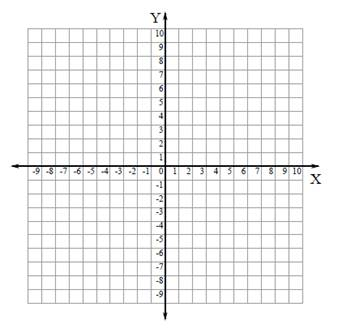# Romeo and Juliet without Shakespeare!

Calculus Level 2On a Chocolate Day , A Mathematician(Romeo) starts from his house which is located at point P(-3,4) and wants reach at His Girlfriend(Juliet) House which is located at point Q(0,1). But instantly he think that he Should buy chocolates for his Girlfriend(Juliet) to impress her. So He went to shop which is located at coordinate X-axis at point R($\alpha$.,0) .But he keep in mind that he should reach to his Girlfriend's House by travelling minimum distance otherwise She will be angry. As he is an Mathematician so he Chose a particular shop so that he reach His Girlfriend's House by travelling minimum Distance.Then find the value of $\alpha$. so that he will impress his Girlfriend.

Let $\alpha$=$\frac { a }{ b }$. Where 'a' and 'b' are coprime integers.

Then find the value of $\quad |a\times b|$.

DETAILS AND ASSUMPTIONS

$\bullet$ Assume that Mathematician is moving in Co-ordinate X-Y plane.

$\bullet$ There are infinite number of Shops at Co-ordinate X-axis.So Mathematician(Romeo) choose Particular Shop To impress his Girlfriend(Juliet).

$\bullet$ Treat all object as point object.

$\bullet$ May be Fermat's Principle of Light waves Helpful Here .

×

Problem Loading...

Note Loading...

Set Loading...